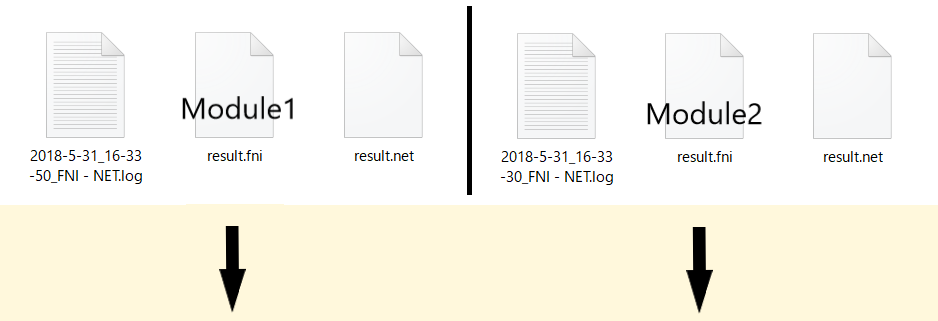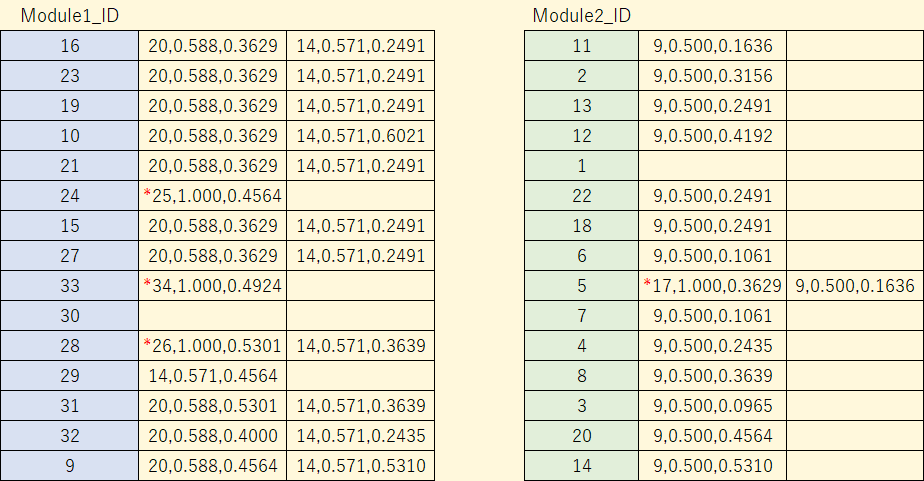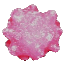# ConfeitoGUI

## ★ Example 4

### ♦ Zachary's karate club

• This social network model was introduced by Zachary WW (J Anthropol Res 1977).
• In the model, 34 members are belonged.
• In a network expressing the model, nodes represent the members and are connected on the basis of their friendships.

### ♦ A dataset for this example

• The below list shows the friendships between the 34 members (edges between the members) based on the Wikipedia page

•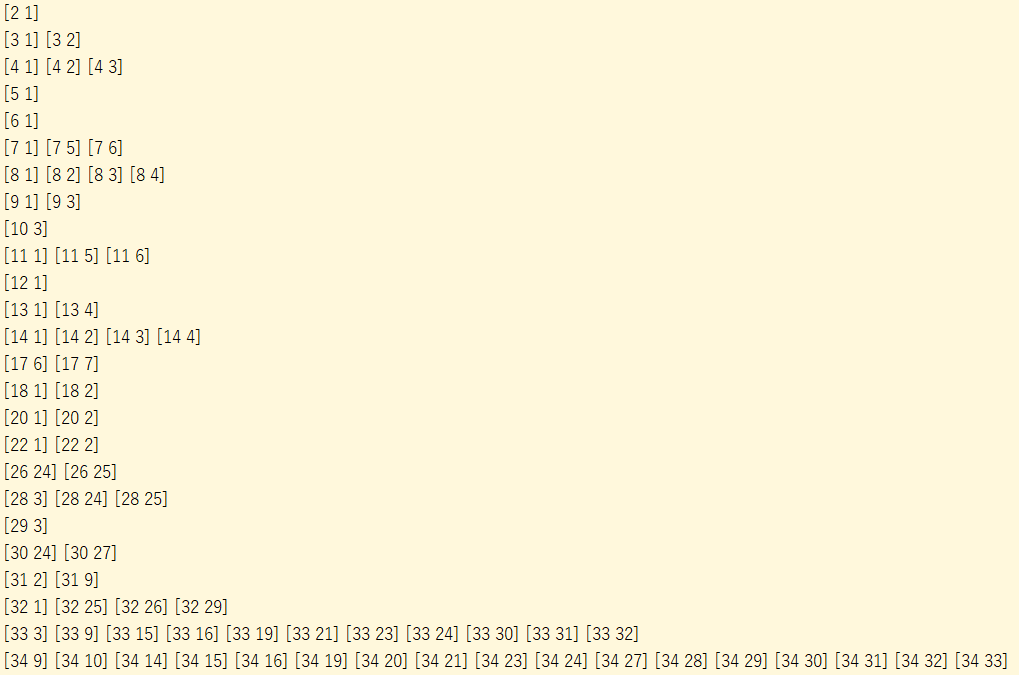• The list was transformed into a matrix containing 34 rows and columns representing the members.
• In the matrix, 1 and 0 represent with and without edges, respectively.

•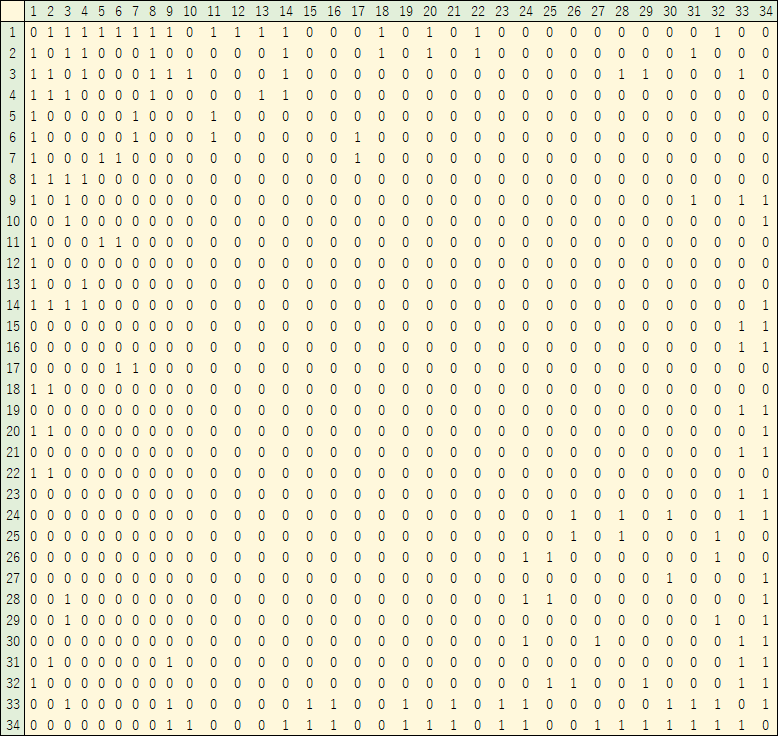• The below network was depicted on the basis of their friendships.
• Circle ( ○ ) and square ( □ ) nodes represent the members of groups (or network modules) separated into two (Module 1 & Module 2), respectively.
• In the circle- and square-marked modules, Nos. 33 and 34 and Nos. 1 and 2 are central members, respectively.
• Based on the network, 12 members (Nos. 1, 2, 3, 9, 10, 14, 20, 28, 31, 32, 33, and 34) have friends in the both modules.
•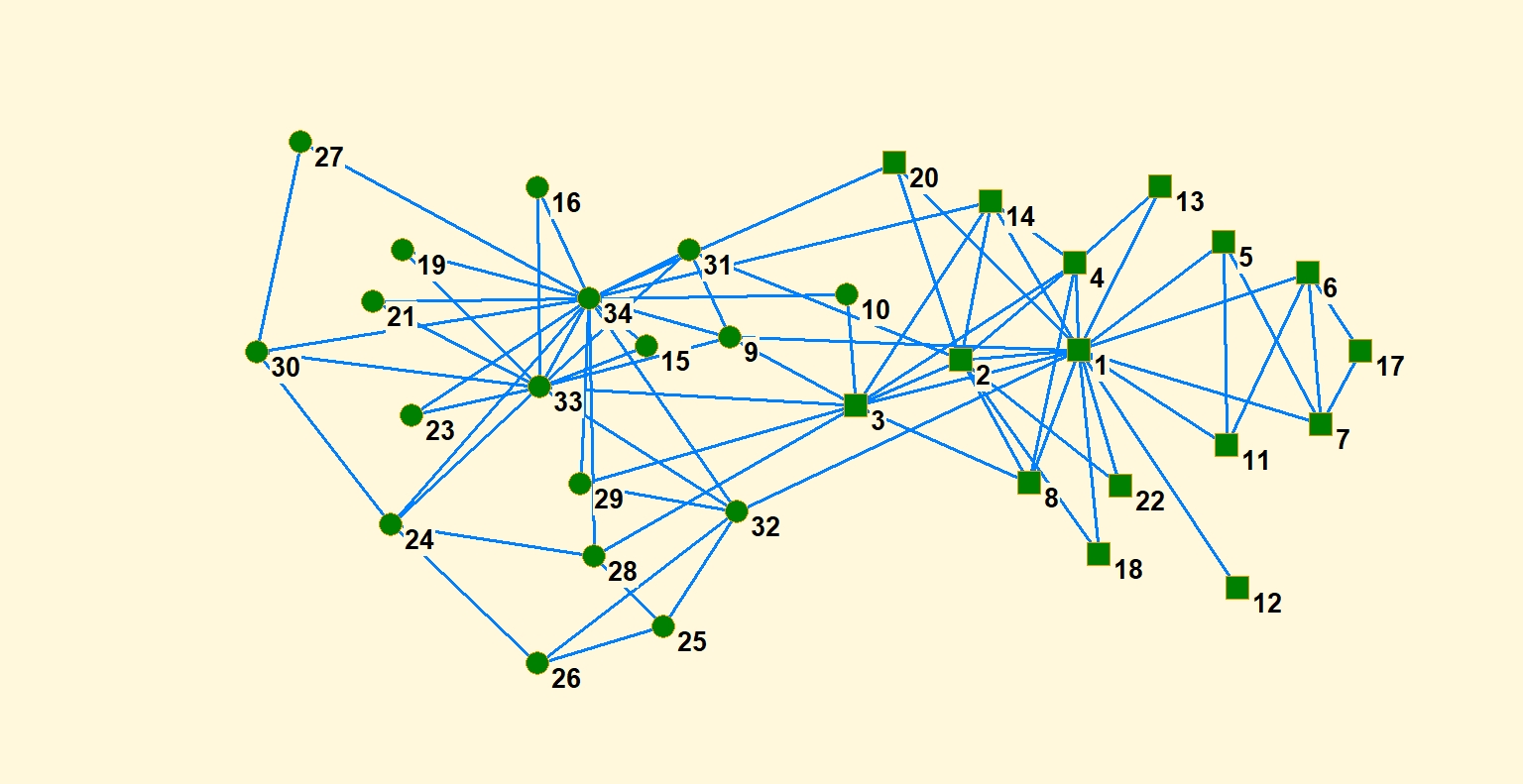• ConfeitoGUI was started. As a type of correlation, Pearson correlation was selected.

•• FPO series was executed.
• In the “FPO (Pearson)” window, “Min and Max Elements” were set as 2 and 30, respectively.

•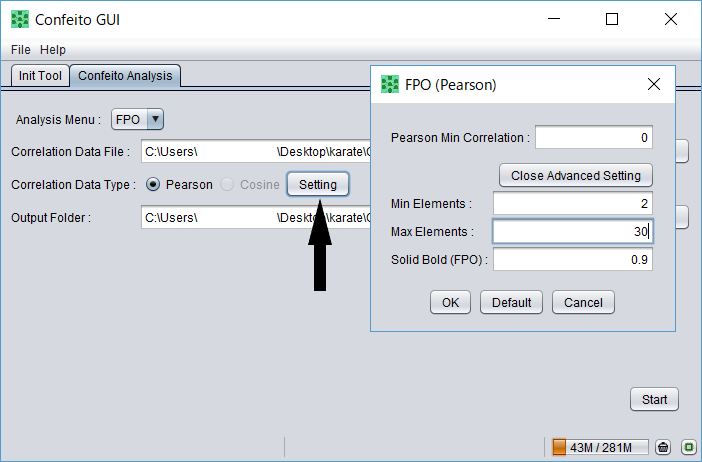• The result of FPO, in which four files are contained.
• A file with an extension of “.net” is available for depicting a network using the Pajek software.

•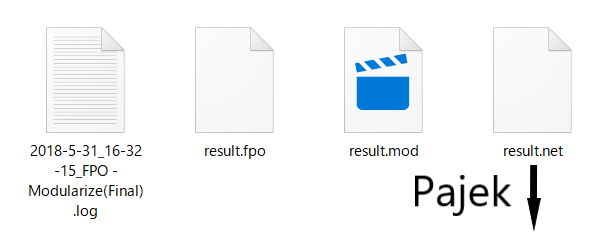• A network of the result was depicted using Pajek.
• In the network, four nodes represented by diamonds (Nos. 17, 25, 26, & 34) were not contained the both modules.
•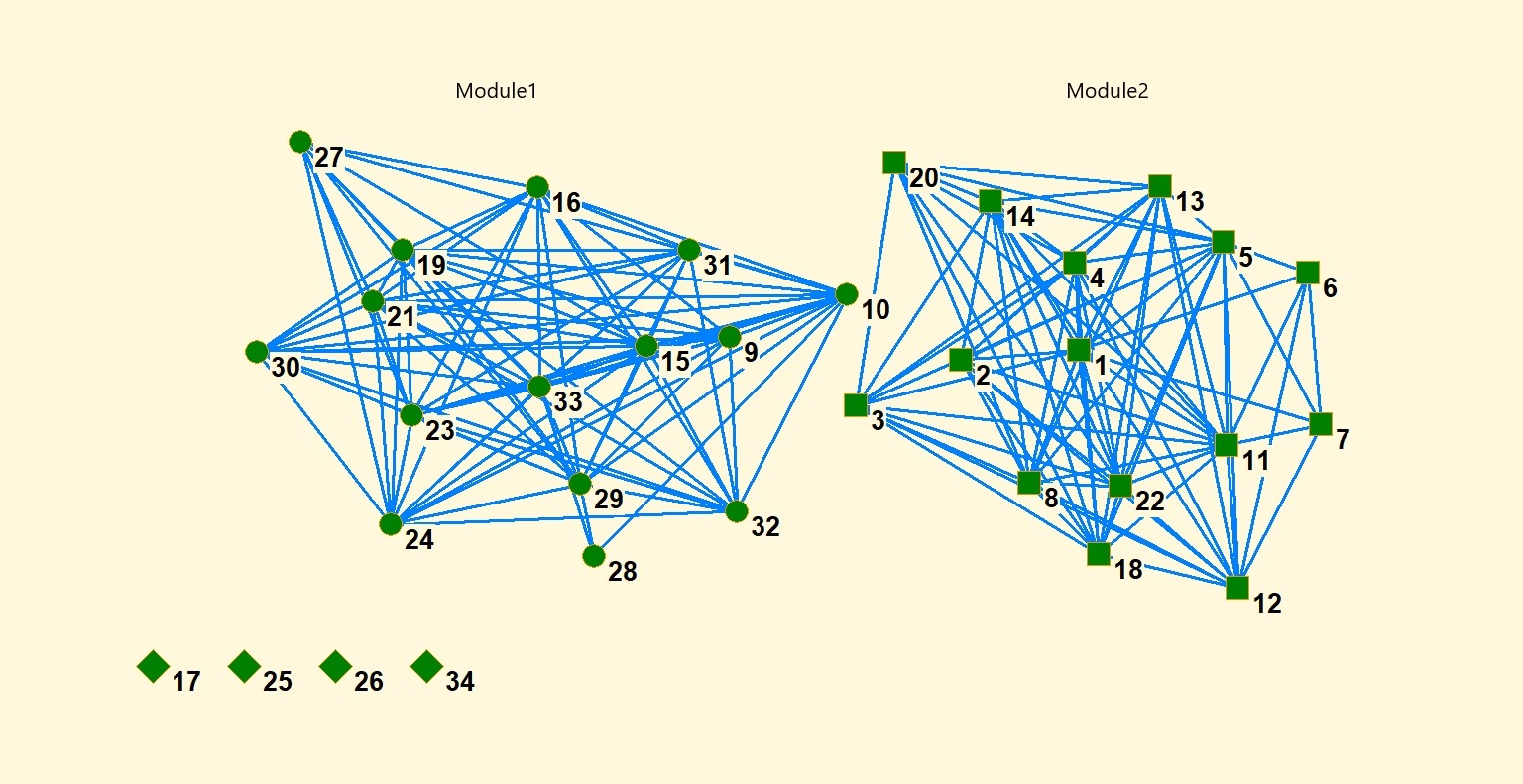• FNI series was executed for the residual four nodes.
• In the series, Nos. 1 & 34 was selected as &ldquot;Target Element&rdquot; for each residual node.
• A target element represent a representative member selected arbitrarily from each module.
• Through the series, it is determined that each residual node has more affinity (or friendliness) to either module.

•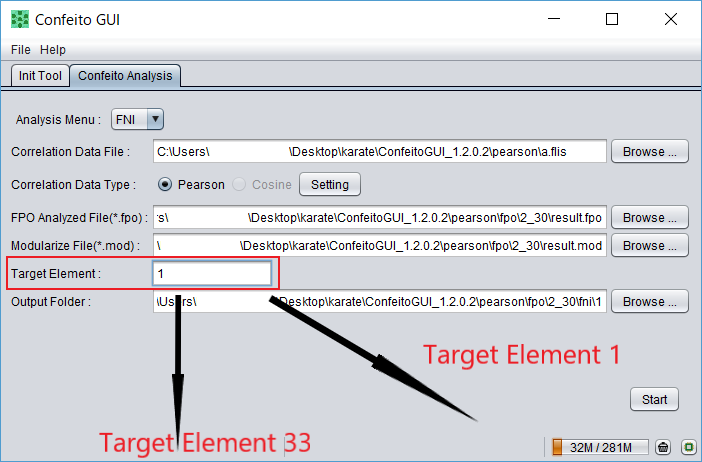• The result of FNI, in which three files are contained for each series; i.e., totally six files for each residual node.
• Through FNI series, a node is assigned to a module.
• Then, the node is connected to a module member with the highest correlation to the residual node.
• In the present case, No. 17 was connected to No. 5; i.e., the node was assigned to the circle-marked module (Module 1).
• Nos. 25, 26, and 34 were connected to No. 24, No. 28, and No. 33, respectively.
• Namely, these residual nodes were assigned to the square-marked module (Module 2).
• In conclusion, all of the members were assigned to the right groups.

•